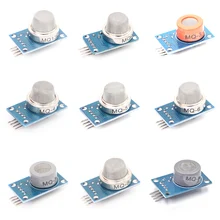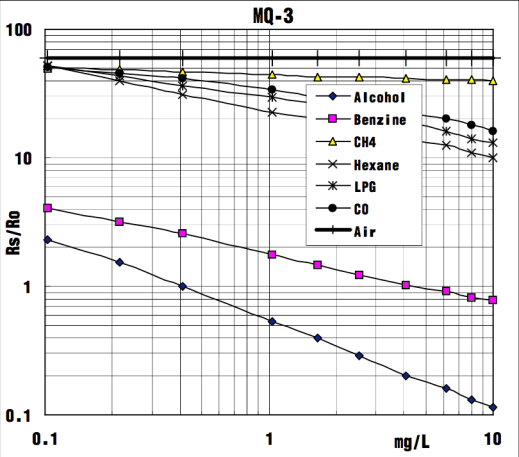Home Arduino Arduino and MQ-3 Gas sensor

# Arduino and MQ-3 Gas sensor

THE MQ-3 is suitable for detecting Alcohol, Benzine, CH4, Hexane, LPG, CO. The sensitivity of the sensor can be adjusted by using the potentiometer.

FEATURES

* High sensitivity to alcohol and small sensitivity to Benzine .
* Fast response and High sensitivity
* Stable and long life
* Simple drive circuit

APPLICATION

They are suitable for alcohol checker, Breathalyser.

There are a lot of these modules – here is an image of some of them### Schematic

Here is a schematic of the module### Connection

Arduino Gas Sensor
5V VCC
GND GND
NC NC
Analog A0 SIG

### Code

To build a reliable alcohol level sensor using the MQ-3, we need to determine the R0 of the device first. We can use this sketch for that:

Basic example

```void setup()
{
Serial.begin(9600);
}

void loop()
{
float sensorVolt;
float sensorValue;

sensorVolt = sensorValue/1024*5.0;

Serial.print("sensor voltage = ");
Serial.print(sensorVolt);
Serial.println("V");
delay(1000);
}```

To build a reliable alcohol level sensor using the MQ-3, we need to determine the R0 of the device first. We can use this sketch for that:

```void setup()
{
Serial.begin(9600);
}

void loop()
{
float sensor_volt;
float RS; // Get the value of RS via in a clear air
float R0; // Get the value of R0 via in Alcohol
float sensorValue;

for(int i = 0 ; i < 100 ; i++)
{
}

sensorValue = sensorValue/100.0; //get average of reading
sensor_volt = sensorValue/1024*5.0;
RS = (5.0-sensor_volt)/sensor_volt; //
R0 = RS/60.0; // 60 is found using interpolation
Serial.print("R0 = ");
Serial.println(R0);
delay(1000);

}```

Open the serial monitor of Arduino IDE. Write down the value of R0 – this is required for the next sketch example. Please write down the R0 after the reading stabilizes.
Replace the R0 below with value of R0 tested above . Expose the sensor to any one of the gases listed at the start.

```void setup()
{
Serial.begin(9600);
}

void loop()
{
float sensor_volt;
float RS_gas; // Get value of RS in a GAS
float ratio; // Get ratio RS_GAS/RS_air
float BAC;
sensor_volt=(float)sensorValue/1024*5.0;
RS_gas = (5.0-sensor_volt)/sensor_volt; // omit *RL

/*-Replace "R0" with the value of R0 with the value earlier*/
ratio = RS_gas/R0; // ratio = RS/R0
BAC = 0.1896*ratio^2 - 8.6178*ratio/10 + 1.0792 //BAC in mg/L
Serial.print("BAC = ");
Serial.println(BAC*0.0001); //convert to g/dL
Serial.print("\n\n");
delay(1000);
}```

MQ-3